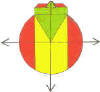DMD   EDU-HOME

WELCOME TO THIS PAGE INTENDED FOR STUDENTS AT THE UNIVERSITY OF THE WESTERN CAPE REGISTERED IN 2010  FOR THE MODULE:

QSF  132

T1 W4 L1

• TUT GROUP 1
• TUTS A7 and A8
•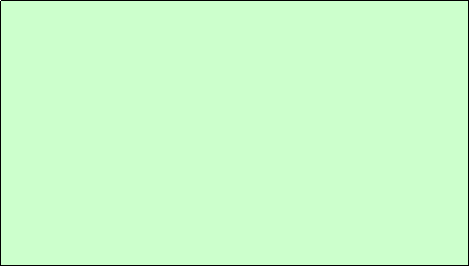CLASS TEST 1 : THURS 5 AUG 2010

BASED ON:

1.     UNIT 1:

·                     VAT (WASHING MACHINE)

·                     POPULATION

·                     2=1???? (p.44)

2.     TUTS A1 to A6

3.     TUTORIAL TEST 1

TODAY:

1.       HISTORY OF THE DECIMAL NUMBER SYSTEM

2.       ROMAN SYSTEM

3.       HINDU-ARABIC SYSTEM

4.       PLACE VALUE & NOTATION

5.       BINARY NUMBERS:

This means that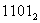= 1 × 2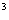+  1 × 2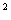+  0 ×  2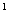+   1 × 2which works out to 8 + 4 + 0 + 1 = 13  in the decimal number system

6.       CONVERSIONS

DEC to BIN : SUCCESSIVE DIVISION

BIN TO DEC : EXPANDED NOTATION+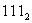=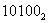8.       SUBTRACTION : THE SHOP METHOD

9.       MULTIPLICATION

10.     DIVISION

11

2 .  NUMBER SYSTEM

2.4  Real Numbers

The following diagram illustrates the connections between the various types of numbers: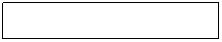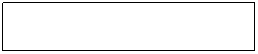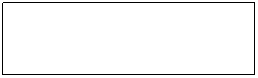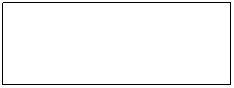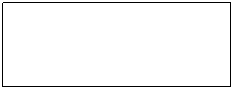INTEGERS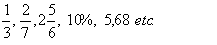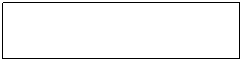OTHER FRACTIONS:

Natural numbers form the most common set of numbers.  They are:

1,2,3, and so on.   It is convenient to write them in set notation form (refer to 2.4.1 below): N = {1;2;3;…}.   When we add the element 0 to the set N, we now have the set of counting numbers, often denoted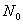.    By adding the negative numbers 0-1,-2,-3,  and so on, to N, we form the set of integers or whole numbers often denoted Z.

The set of integers or whole numbers, often denoted by  Z or rarely I (for Integers), can be represented on a line, called the real number line:

-4       -3         -2         -1           0          1          2          3           4

.           .                .                .        .       .       .      .             .

It can be represented in set form as:   Z = {0;+1;-1;+2;-2;+3;-3:…} or

Z = {...;-3;-2;-1;0;1;2;3;…}.

Real numbers (denoted R) consists of two types of numbers: rational numbers (denoted Q) and irrational numbers (denoted I).  Rational numbers are those numbers, which can be represented in the form of a fraction  a/b, where a and b are integers.  Clearly all integers are rational numbers because any integer can be written in the form of a fraction: that integer/1.  However, there are infinitely many rational numbers on the number line, and even between 0 and 1.

In QSF 132 you will have to know fractions very well, as well as related forms of decimal fractions and percentages. In your business-related problems in SQF, you will need to have a thorough understanding of these, and particularly of operations on them such as addition and multiplication  These three (fractions, decimal fractions and percentages) can all be written in the form of a fraction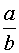, and are therefore rational numbers.

Irrational numbers are ones that cannot be written in the form of a fraction.  There are also infinitely many of them.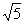is an example. You have probably tried to find the square root of a number which cannot be determined exactly to a fixed number of decimal places.

Although a calculator gives 1/3 as 0.3333333333.....  which is a number with decimals that recur infinitely many times; ⅓, however, is a rational number.  An irrational number has decimals which have no discernable pattern.  So when you work with you calculator you must not think that because the decimals never seems to end, that it is an irrational number!  Sometimes it is a rational number such as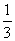,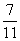, and so on. Try to convert these rational numbers to decimal numbers using your calculator, and conversely.  You will notice that the decimal part is in a recurring pattern. (= 0.636363… .).

If you are required to show that 2.51, for example, is a rational number, you have to show something as  follows: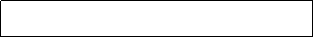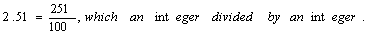NB!!!!!!

12. SETS

60 Tourists stayed at a resort hotel.  Of them, 37 stayed for at least one week, 43 spent at least R500 a day, while 32 were completely satisfied with their accommodation. Furthermore, 30 stayed for a least one week and spent at least R500 a day, 26 stayed for at least one week and were completely satisfied with their accommodation, whereas 27 spent at least R500 a day and were completely satisfied with their accommodation. 24 stayed for at least one week, spent at least R500 a day and were completely satisfied with their accommodation.

(a)               How many visitors stayed for at least one week, spent at least R500 a day but were not completely satisfied with their accommodation? (Ans 6)

(b)               How many visitors were completely satisfied with their accommodation, but stayed less than one week and spent less than R500 a day? (Ans 3)

(c)               How many visitors stayed less than one week, spent less than R500 a day and were not completely satisfied with their accommodation? (Ans 7)

©  DESMOND DESAI, DMD EDU-HOME, 2010

All rights reserved. Designed and created by Desmond Desai, South Africa. This page is protected by Copyright. No part of this page may be reproduced, stored in a retrieval system, or transmitted in any form of by any means, electronic, mechanical, photocopying, recording or otherwise, without the prior written permission of the copyright holder.